# 3.1 Defining the derivative  (Page 6/10)

 Page 6 / 10

$f\left(x\right)=4x+7;{x}_{1}=2,{x}_{2}=5$

$4$

$f\left(x\right)=8x-3;{x}_{1}=-1,{x}_{2}=3$

$f\left(x\right)={x}^{2}+2x+1;{x}_{1}=3,{x}_{2}=3.5$

$8.5$

$f\left(x\right)=\text{−}{x}^{2}+x+2;{x}_{1}=0.5,{x}_{2}=1.5$

$f\left(x\right)=\frac{4}{3x-1};{x}_{1}=1,{x}_{2}=3$

$-\frac{3}{4}$

$f\left(x\right)=\frac{x-7}{2x+1};{x}_{1}=-2,{x}_{2}=0$

$f\left(x\right)=\sqrt{x};{x}_{1}=1,{x}_{2}=16$

$0.2$

$f\left(x\right)=\sqrt{x-9};{x}_{1}=10,{x}_{2}=13$

$f\left(x\right)={x}^{1\text{/}3}+1;{x}_{1}=0,{x}_{2}=8$

$0.25$

$f\left(x\right)=6{x}^{2\text{/}3}+2{x}^{1\text{/}3};{x}_{1}=1,{x}_{2}=27$

For the following functions,

1. use [link] to find the slope of the tangent line ${m}_{\text{tan}}={f}^{\prime }\left(a\right),$ and
2. find the equation of the tangent line to $f$ at $x=a.$

$f\left(x\right)=3-4x,a=2$

a. $-4$ b. $y=3-4x$

$f\left(x\right)=\frac{x}{5}+6,a=-1$

$f\left(x\right)={x}^{2}+x,a=1$

a. $3$ b. $y=3x-1$

$f\left(x\right)=1-x-{x}^{2},a=0$

$f\left(x\right)=\frac{7}{x},a=3$

a. $\frac{-7}{9}$ b. $y=\frac{-7}{9}x+\frac{14}{3}$

$f\left(x\right)=\sqrt{x+8},a=1$

$f\left(x\right)=2-3{x}^{2},a=-2$

a. $12$ b. $y=12x+14$

$f\left(x\right)=\frac{-3}{x-1},a=4$

$f\left(x\right)=\frac{2}{x+3},a=-4$

a. $-2$ b. $y=-2x-10$

$f\left(x\right)=\frac{3}{{x}^{2}},a=3$

For the following functions $y=f\left(x\right),$ find ${f}^{\prime }\left(a\right)$ using [link] .

$f\left(x\right)=5x+4,a=-1$

$5$

$f\left(x\right)=-7x+1,a=3$

$f\left(x\right)={x}^{2}+9x,a=2$

$13$

$f\left(x\right)=3{x}^{2}-x+2,a=1$

$f\left(x\right)=\sqrt{x},a=4$

$\frac{1}{4}$

$f\left(x\right)=\sqrt{x-2},a=6$

$f\left(x\right)=\frac{1}{x},a=2$

$-\frac{1}{4}$

$f\left(x\right)=\frac{1}{x-3},a=-1$

$f\left(x\right)=\frac{1}{{x}^{3}},a=1$

$-3$

$f\left(x\right)=\frac{1}{\sqrt{x}},a=4$

For the following exercises, given the function $y=f\left(x\right),$

1. find the slope of the secant line $PQ$ for each point $Q\left(x,f\left(x\right)\right)$ with $x$ value given in the table.
2. Use the answers from a. to estimate the value of the slope of the tangent line at $P.$
3. Use the answer from b. to find the equation of the tangent line to $f$ at point $P.$

[T] $f\left(x\right)={x}^{2}+3x+4,P\left(1,8\right)$ (Round to $6$ decimal places.)

x Slope ${m}_{PQ}$ x Slope ${m}_{PQ}$
1.1 (i) 0.9 (vii)
1.01 (ii) 0.99 (viii)
1.001 (iii) 0.999 (ix)
1.0001 (iv) 0.9999 (x)
1.00001 (v) 0.99999 (xi)
1.000001 (vi) 0.999999 (xii)

a. $\text{(i)}\phantom{\rule{0.2em}{0ex}}5.100000,$ $\text{(ii)}\phantom{\rule{0.2em}{0ex}}5.010000,$ $\text{(iii)}\phantom{\rule{0.2em}{0ex}}5.001000,$ $\text{(iv)}\phantom{\rule{0.2em}{0ex}}5.000100,$ $\text{(v)}\phantom{\rule{0.2em}{0ex}}5.000010,$ $\text{(vi)}\phantom{\rule{0.2em}{0ex}}5.000001,$ $\text{(vii)}\phantom{\rule{0.2em}{0ex}}4.900000,$ $\text{(viii)}\phantom{\rule{0.2em}{0ex}}4.990000,$ $\text{(ix)}\phantom{\rule{0.2em}{0ex}}4.999000,$ $\text{(x)}\phantom{\rule{0.2em}{0ex}}4.999900,$ $\text{(xi)}\phantom{\rule{0.2em}{0ex}}4.999990,$ $\text{(x)}\phantom{\rule{0.2em}{0ex}}4.999999$ b. ${m}_{\text{tan}}=5$ c. $y=5x+3$

[T] $f\left(x\right)=\frac{x+1}{{x}^{2}-1},P\left(0,-1\right)$

x Slope ${m}_{PQ}$ x Slope ${m}_{PQ}$
0.1 (i) $-0.1$ (vii)
0.01 (ii) $-0.01$ (viii)
0.001 (iii) $-0.001$ (ix)
0.0001 (iv) $-0.0001$ (x)
0.00001 (v) $-0.00001$ (xi)
0.000001 (vi) $-0.000001$ (xii)

[T] $f\left(x\right)=10{e}^{0.5x},P\left(0,10\right)$ (Round to $4$ decimal places.)

x Slope ${m}_{PQ}$
$-0.1$ (i)
$-0.01$ (ii)
$-0.001$ (iii)
$-0.0001$ (iv)
$-0.00001$ (v)
−0.000001 (vi)

a. $\text{(i)}\phantom{\rule{0.2em}{0ex}}4.8771,$ $\text{(ii)}\phantom{\rule{0.2em}{0ex}}4.9875\phantom{\rule{0.2em}{0ex}}\text{(iii)}\phantom{\rule{0.2em}{0ex}}4.9988,$ $\text{(iv)}\phantom{\rule{0.2em}{0ex}}4.9999,$ $\text{(v)}\phantom{\rule{0.2em}{0ex}}4.9999,$ $\text{(vi)}\phantom{\rule{0.2em}{0ex}}4.9999$ b. ${m}_{\text{tan}}=5$ c. $y=5x+10$

[T] $f\left(x\right)=\text{tan}\phantom{\rule{0.1em}{0ex}}\left(x\right),P\left(\pi ,0\right)$

x Slope ${m}_{PQ}$
3.1 (i)
3.14 (ii)
3.141 (iii)
3.1415 (iv)
3.14159 (v)
3.141592 (vi)

[T] For the following position functions $y=s\left(t\right),$ an object is moving along a straight line, where $t$ is in seconds and $s$ is in meters. Find

1. the simplified expression for the average velocity from $t=2$ to $t=2+h;$
2. the average velocity between $t=2$ and $t=2+h,$ where $\text{(i)}\phantom{\rule{0.2em}{0ex}}h=0.1,$ $\text{(ii)}\phantom{\rule{0.2em}{0ex}}h=0.01,$ $\text{(iii)}\phantom{\rule{0.2em}{0ex}}h=0.001,$ and $\text{(iv)}\phantom{\rule{0.2em}{0ex}}h=0.0001;$ and
3. use the answer from a. to estimate the instantaneous velocity at $t=2$ second.

$s\left(t\right)=\frac{1}{3}t+5$

a. $\frac{1}{3};$ b. $\text{(i)}\phantom{\rule{0.2em}{0ex}}0.\stackrel{–}{3}$ m/s, $\text{(ii)}\phantom{\rule{0.2em}{0ex}}0.\stackrel{–}{3}$ m/s, $\text{(iii)}\phantom{\rule{0.2em}{0ex}}0.\stackrel{–}{3}$ m/s, $\text{(iv)}\phantom{\rule{0.2em}{0ex}}0.\stackrel{–}{3}$ m/s; c. $0.\stackrel{–}{3}=\frac{1}{3}$ m/s

$s\left(t\right)={t}^{2}-2t$

$s\left(t\right)=2{t}^{3}+3$

a. $2\left({h}^{2}+6h+12\right);$ b. $\text{(i)}\phantom{\rule{0.2em}{0ex}}25.22$ m/s, $\text{(ii)}\phantom{\rule{0.2em}{0ex}}24.12$ m/s, $\text{(iii)}\phantom{\rule{0.2em}{0ex}}24.01$ m/s, $\text{(iv)}\phantom{\rule{0.2em}{0ex}}24$ m/s; c. $24$ m/s

$s\left(t\right)=\frac{16}{{t}^{2}}-\frac{4}{t}$

Use the following graph to evaluate a. ${f}^{\prime }\left(1\right)$ and b. ${f}^{\prime }\left(6\right).$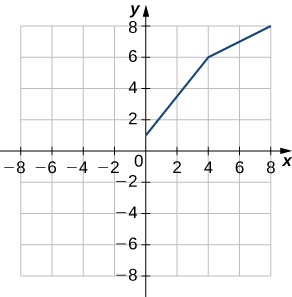a. $1.25;$ b. $0.5$

Use the following graph to evaluate a. ${f}^{\prime }\left(-3\right)$ and b. ${f}^{\prime }\left(1.5\right).$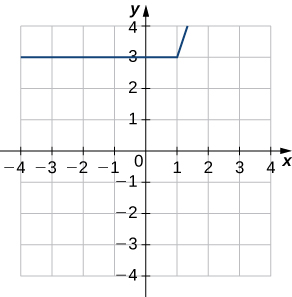For the following exercises, use the limit definition of derivative to show that the derivative does not exist at $x=a$ for each of the given functions.

$f\left(x\right)={x}^{1\text{/}3},x=0$

$\underset{x\to {0}^{-}}{\text{lim}}\frac{{x}^{1\text{/}3}-0}{x-0}=\underset{x\to {0}^{-}}{\text{lim}}\frac{1}{{x}^{2\text{/}3}}=\infty$

$f\left(x\right)={x}^{2\text{/}3},x=0$

$f\left(x\right)=\left\{\begin{array}{c}1,x<1\\ x,x\ge 1\end{array},x=1$

$\underset{x\to {1}^{-}}{\text{lim}}\frac{1-1}{x-1}=0\ne 1=\underset{x\to {1}^{+}}{\text{lim}}\frac{x-1}{x-1}$

$f\left(x\right)=\frac{|x|}{x},x=0$

[T] The position in feet of a race car along a straight track after $t$ seconds is modeled by the function $s\left(t\right)=8{t}^{2}-\frac{1}{16}{t}^{3}.$

1. Find the average velocity of the vehicle over the following time intervals to four decimal places:
1. [4, 4.1]
2. [4, 4.01]
3. [4, 4.001]
4. [4, 4.0001]
2. Use a. to draw a conclusion about the instantaneous velocity of the vehicle at $t=4$ seconds.

a. $\text{(i)}\phantom{\rule{0.2em}{0ex}}61.7244$ ft/s, $\text{(ii)}\phantom{\rule{0.2em}{0ex}}61.0725$ ft/s $\text{(iii)}\phantom{\rule{0.2em}{0ex}}61.0072$ ft/s $\text{(iv)}\phantom{\rule{0.2em}{0ex}}61.0007$ ft/s b. At $4$ seconds the race car is traveling at a rate/velocity of $61$ ft/s.

[T] The distance in feet that a ball rolls down an incline is modeled by the function $s\left(t\right)=14{t}^{2},$ where t is seconds after the ball begins rolling.

1. Find the average velocity of the ball over the following time intervals:
1. [5, 5.1]
2. [5, 5.01]
3. [5, 5.001]
4. [5, 5.0001]
2. Use the answers from a. to draw a conclusion about the instantaneous velocity of the ball at $t=5$ seconds.

Two vehicles start out traveling side by side along a straight road. Their position functions, shown in the following graph, are given by $s=f\left(t\right)$ and $s=g\left(t\right),$ where $s$ is measured in feet and $t$ is measured in seconds.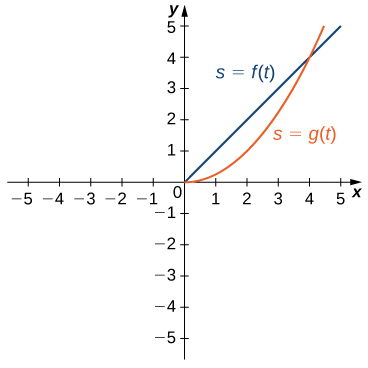1. Which vehicle has traveled farther at $t=2$ seconds?
2. What is the approximate velocity of each vehicle at $t=3$ seconds?
3. Which vehicle is traveling faster at $t=4$ seconds?
4. What is true about the positions of the vehicles at $t=4$ seconds?

a. The vehicle represented by $f\left(t\right),$ because it has traveled $2$ feet, whereas $g\left(t\right)$ has traveled $1$ foot. b. The velocity of $f\left(t\right)$ is constant at $1$ ft/s, while the velocity of $g\left(t\right)$ is approximately $2$ ft/s. c. The vehicle represented by $g\left(t\right),$ with a velocity of approximately $4$ ft/s. d. Both have traveled $4$ feet in $4$ seconds.

[T] The total cost $C\left(x\right),$ in hundreds of dollars, to produce $x$ jars of mayonnaise is given by $C\left(x\right)=0.000003{x}^{3}+4x+300.$

1. Calculate the average cost per jar over the following intervals:
1. [100, 100.1]
2. [100, 100.01]
3. [100, 100.001]
4. [100, 100.0001]
2. Use the answers from a. to estimate the average cost to produce $100$ jars of mayonnaise.

[T] For the function $f\left(x\right)={x}^{3}-2{x}^{2}-11x+12,$ do the following.

1. Use a graphing calculator to graph f in an appropriate viewing window.
2. Use the ZOOM feature on the calculator to approximate the two values of $x=a$ for which ${m}_{\text{tan}}={f}^{\prime }\left(a\right)=0.$

a.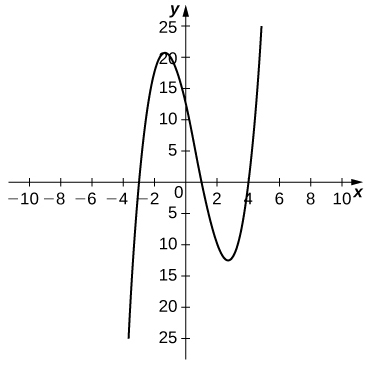b. $a\approx -1.361,2.694$

[T] For the function $f\left(x\right)=\frac{x}{1+{x}^{2}},$ do the following.

1. Use a graphing calculator to graph $f$ in an appropriate viewing window.
2. Use the ZOOM feature on the calculator to approximate the values of $x=a$ for which ${m}_{\text{tan}}={f}^{\prime }\left(a\right)=0.$

Suppose that $N\left(x\right)$ computes the number of gallons of gas used by a vehicle traveling $x$ miles. Suppose the vehicle gets $30$ mpg.

1. Find a mathematical expression for $N\left(x\right).$
2. What is $N\left(100\text{)?}$ Explain the physical meaning.
3. What is ${N}^{\prime }\left(100\right)?$ Explain the physical meaning.

a. $N\left(x\right)=\frac{x}{30}$ b. $\sim 3.3$ gallons. When the vehicle travels $100$ miles, it has used $3.3$ gallons of gas. c. $\frac{1}{30}.$ The rate of gas consumption in gallons per mile that the vehicle is achieving after having traveled $100$ miles.

[T] For the function $f\left(x\right)={x}^{4}-5{x}^{2}+4,$ do the following.

1. Use a graphing calculator to graph $f$ in an appropriate viewing window.
2. Use the $\text{nDeriv}$ function, which numerically finds the derivative, on a graphing calculator to estimate ${f}^{\prime }\left(-2\right),{f}^{\prime }\left(-0.5\right),{f}^{\prime }\left(1.7\right),$ and ${f}^{\prime }\left(2.718\right).$

[T] For the function $f\left(x\right)=\frac{{x}^{2}}{{x}^{2}+1},$ do the following.

1. Use a graphing calculator to graph $f$ in an appropriate viewing window.
2. Use the $\text{nDeriv}$ function on a graphing calculator to find ${f}^{\prime }\left(-4\right),{f}^{\prime }\left(-2\right),{f}^{\prime }\left(2\right),$ and ${f}^{\prime }\left(4\right).$

a.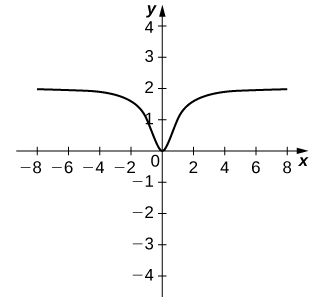b. $-0.028,-0.16,0.16,0.028$

f(x) = x-2 g(x) = 3x + 5 fog(x)? f(x)/g(x)
fog(x)= f(g(x)) = x-2 = 3x+5-2 = 3x+3 f(x)/g(x)= x-2/3x+5
diron
pweding paturo nsa calculus?
jimmy
how to use fundamental theorem to solve exponential
find the bounded area of the parabola y^2=4x and y=16x
what is absolute value means?
Chicken nuggets
Hugh
🐔
MM
🐔🦃 nuggets
MM
(mathematics) For a complex number a+bi, the principal square root of the sum of the squares of its real and imaginary parts, √a2+b2 . Denoted by | |. The absolute value |x| of a real number x is √x2 , which is equal to x if x is non-negative, and −x if x is negative.
Ismael
find integration of loge x
find the volume of a solid about the y-axis, x=0, x=1, y=0, y=7+x^3
how does this work
Can calculus give the answers as same as other methods give in basic classes while solving the numericals?
log tan (x/4+x/2)
Rohan
Rohan
y=(x^2 + 3x).(eipix)
Claudia
Ismael
A Function F(X)=Sinx+cosx is odd or even?
neither
David
Neither
Lovuyiso
f(x)=1/1+x^2 |=[-3,1]
apa itu?
fauzi
determine the area of the region enclosed by x²+y=1,2x-y+4=0
Hi
MP
Hi too
Vic
hello please anyone with calculus PDF should share
Which kind of pdf do you want bro?
Aftab
hi
Abdul
can I get calculus in pdf
Abdul
explain for me
Usman
okay I have such documents
Fitzgerald
Hamza
How to use it to slove fraction
Hello please can someone tell me the meaning of this group all about, yes I know is calculus group but yet nothing is showing up
Shodipo
You have downloaded the aplication Calculus Volume 1, tackling about lessons for (mostly) college freshmen, Calculus 1: Differential, and this group I think aims to let concerns and questions from students who want to clarify something about the subject. Well, this is what I guess so.
Jean
Im not in college but this will still help
nothing
how en where can u apply it
Migos
how can we scatch a parabola graph
Ok
Endalkachew
how can I solve differentiation?
with the help of different formulas and Rules. we use formulas according to given condition or according to questions
CALCULUS
For example any questions...
CALCULUS
v=(x,y) وu=(x,y ) ∂u/∂x* ∂x/∂u +∂v/∂x*∂x/∂v=1
log tan (x/4+x/2)
Rohan
what is the procedures in solving number 1?

#### Get Jobilize Job Search Mobile App in your pocket Now!By Cath YuBy OpenStaxBy OpenStaxBy Olivia D'AmbrogioBy OpenStaxBy OpenStaxBy OpenStaxByByBy Brenna Fike